# Resources tagged with: Place value

Filter by: Content type:
Age range:
Challenge level:

### There are 91 results

Broad Topics > The Number System and Place Value > Place value### Napier's Bones

##### Age 7 to 11Challenge Level

The Scot, John Napier, invented these strips about 400 years ago to help calculate multiplication and division. Can you work out how to use Napier's bones to find the answer to these multiplications?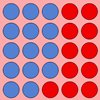### Place Value as a Building Block for Developing Fluency in the Calculation Process

##### Age 5 to 11

This article for primary teachers encourages exploration of two fundamental ideas, exchange and 'unitising', which will help children become more fluent when calculating.### One Million to Seven

##### Age 7 to 11Challenge Level

Start by putting one million (1 000 000) into the display of your calculator. Can you reduce this to 7 using just the 7 key and add, subtract, multiply, divide and equals as many times as you like?### Six Is the Sum

##### Age 7 to 11Challenge Level

What do the digits in the number fifteen add up to? How many other numbers have digits with the same total but no zeros?### Spell by Numbers

##### Age 7 to 11Challenge Level

Can you substitute numbers for the letters in these sums?### Being Resilient - Primary Number

##### Age 5 to 11Challenge Level

Number problems at primary level that may require resilience.### Being Resourceful - Primary Number

##### Age 5 to 11Challenge Level

Number problems at primary level that require careful consideration.### ABC

##### Age 7 to 11Challenge Level

In the multiplication calculation, some of the digits have been replaced by letters and others by asterisks. Can you reconstruct the original multiplication?### Reach 100

##### Age 7 to 11Challenge Level

Choose four different digits from 1-9 and put one in each box so that the resulting four two-digit numbers add to a total of 100.### All the Digits

##### Age 7 to 11Challenge Level

This multiplication uses each of the digits 0 - 9 once and once only. Using the information given, can you replace the stars in the calculation with figures?### Multiply Multiples 1

##### Age 7 to 11Challenge Level

Can you complete this calculation by filling in the missing numbers? In how many different ways can you do it?### Multiply Multiples 3

##### Age 7 to 11Challenge Level

Have a go at balancing this equation. Can you find different ways of doing it?### Multiply Multiples 2

##### Age 7 to 11Challenge Level

Can you work out some different ways to balance this equation?### Trebling

##### Age 7 to 11Challenge Level

Can you replace the letters with numbers? Is there only one solution in each case?### Alien Counting

##### Age 7 to 11Challenge Level

Investigate the different ways these aliens count in this challenge. You could start by thinking about how each of them would write our number 7.### Oddly

##### Age 7 to 11Challenge Level

Find the sum of all three-digit numbers each of whose digits is odd.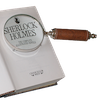### Number Detective

##### Age 5 to 11Challenge Level

Follow the clues to find the mystery number.##### Age 5 to 11Challenge Level

Try out this number trick. What happens with different starting numbers? What do you notice?### Being Collaborative - Primary Number

##### Age 5 to 11Challenge Level

Number problems at primary level to work on with others.### Arrange the Digits

##### Age 11 to 14Challenge Level

Can you arrange the digits 1,2,3,4,5,6,7,8,9 into three 3-digit numbers such that their total is close to 1500?### Journeys on the Gattegno Tens Chart

##### Age 5 to 11

Alf describes how the Gattegno chart helped a class of 7-9 year olds gain an awareness of place value and of the inverse relationship between multiplication and division.### Song Book

##### Age 7 to 11Challenge Level

A school song book contains 700 songs. The numbers of the songs are displayed by combining special small single-digit cards. What is the minimum number of small cards that is needed?### Round the Three Dice

##### Age 7 to 11Challenge Level

What happens when you round these three-digit numbers to the nearest 100?##### Age 11 to 14Challenge Level

By selecting digits for an addition grid, what targets can you make?### Coded Hundred Square

##### Age 7 to 11Challenge Level

This 100 square jigsaw is written in code. It starts with 1 and ends with 100. Can you build it up?##### Age 11 to 14Challenge Level

Visitors to Earth from the distant planet of Zub-Zorna were amazed when they found out that when the digits in this multiplication were reversed, the answer was the same! Find a way to explain. . . .##### Age 5 to 11Challenge Level

Who said that adding couldn't be fun?### Diagonal Sums

##### Age 7 to 14Challenge Level

In this 100 square, look at the green square which contains the numbers 2, 3, 12 and 13. What is the sum of the numbers that are diagonally opposite each other? What do you notice?### Round the Dice Decimals 2

##### Age 7 to 11Challenge Level

What happens when you round these numbers to the nearest whole number?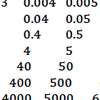### How Can I Support the Development of Early Number Sense and Place Value?

##### Age 3 to 11

This article for primary teachers expands on the key ideas which underpin early number sense and place value, and suggests activities to support learners as they get to grips with these ideas.### Round the Dice Decimals 1

##### Age 7 to 11Challenge Level

Use two dice to generate two numbers with one decimal place. What happens when you round these numbers to the nearest whole number?### Which Is Quicker?

##### Age 7 to 11Challenge Level

Which is quicker, counting up to 30 in ones or counting up to 300 in tens? Why?### Activities on the Gattegno Chart

##### Age 5 to 11

In this article, Alf outlines six activities using the Gattegno chart, which help to develop understanding of place value, multiplication and division.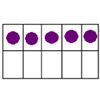### Place Value: the Ten-ness of Ten

##### Age 3 to 11

This article develops the idea of 'ten-ness' as an important element of place value.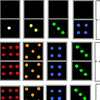### Ordering

##### Age 5 to 11

This set of activities focuses on ordering, an important aspect of place value.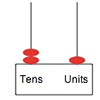### Position

##### Age 5 to 11

One of the key ideas associated with place value is that the position of a digit affects its value. These activities support children in understanding this idea.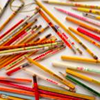### Amount

##### Age 5 to 11

These tasks will help learners develop their understanding of place value, particularly giving them opportunities to express numbers as amounts.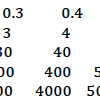### Number Sense and Place Value

##### Age 5 to 11

This feature aims to support you in developing children's early number sense and understanding of place value.### More Upper Primary Activities on Number Sense and Place Value

##### Age 7 to 11

More upper primary number sense and place value tasks.### Nice or Nasty for Two

##### Age 7 to 14Challenge Level

Some Games That May Be Nice or Nasty for an adult and child. Use your knowledge of place value to beat your opponent.##### Age 11 to 14Challenge Level

What happens when you add a three digit number to its reverse?### Nice or Nasty

##### Age 7 to 14Challenge Level

There are nasty versions of this dice game but we'll start with the nice ones...### Subtraction Surprise

##### Age 7 to 14Challenge Level

Try out some calculations. Are you surprised by the results?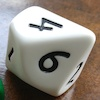### Less Is More

##### Age 5 to 11Challenge Level

Use your knowledge of place value to try to win this game. How will you maximise your score?### The Thousands Game

##### Age 7 to 11Challenge Level

Each child in Class 3 took four numbers out of the bag. Who had made the highest even number?### What Do You Need?

##### Age 7 to 11Challenge Level

Four of these clues are needed to find the chosen number on this grid and four are true but do nothing to help in finding the number. Can you sort out the clues and find the number?### Football Sum

##### Age 11 to 14Challenge Level

Find the values of the nine letters in the sum: FOOT + BALL = GAME##### Age 11 to 14Challenge Level

Replace the letters with numbers to make the addition work out correctly. R E A D + T H I S = P A G E### Repeaters

##### Age 11 to 14Challenge Level

Choose any 3 digits and make a 6 digit number by repeating the 3 digits in the same order (e.g. 594594). Explain why whatever digits you choose the number will always be divisible by 7, 11 and 13.### Legs Eleven

##### Age 11 to 14Challenge Level

Take any four digit number. Move the first digit to the end and move the rest along. Now add your two numbers. Did you get a multiple of 11?